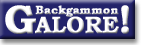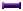TheoryForum Archive : Theory

 Under-doubling dice

 From: Bill Taylor Address: mathwft@math.canterbury.ac.nz Date: 18 December 1997 Subject: Re: Under-doubling dice? Forum: rec.games.backgammon Google: 67ap52\$c1l\$1@cantuc.canterbury.ac.nz

```OK then, having started it, it's my turn to contribute to this thread.

About the matter of using a doubling cube with other games, I'm sure
it's a goer, for money games or long-match games.  The arguments against,
for complete-info games of no chance, (chess etc), struck me as very weak.
Incidentally, I agree that for CI-NC games that are actually *played*,
i.e. for which perfect strategies are as yet unknown, there IS luck in
them, in any reasonable sense of the term.  A chess master pal of mine
from long ago, summed it up well, even profoundly - "In chess there is no
such thing as BAD luck, but there IS good luck".   Rather neat, I thought.

I was particularly struck by the suggestion that a doubling cube be used
for snooker matches, especially on telly - that it would reduce those long
boring near-certain results, and also keep the likely outcome secret
from the program-time-aware viewer.  :)    Also, snooker is quite a high
volatility game, which would make it a natural for a doubling cube.

-----------

However, all that aside, what I really want to talk about here is the
UNDER-doubling part of the thread, for backgammon-style games.
Underdoubling OR OVER-doubling, of course!  A tripling cube might
well be an amusement, especially for the keen gambler.

There's not much in here except a pretty picture, (pretty crumby picture?),
except that I look at the general M-multiplying cube.  So standardly, M=2.

Like others, I *only* look at the very sub-backgammon-like situation
where there is ZERO VOLATILITY.  This is like the tug-o-dice game, only
more so.  We model the game as a diffusion, with a variable moving between
0 and 1, this being the cubeless P(WIN) for one player.  This variable
starts at 0.5, and drifts continuously to and fro on the [0,1] interval,
with equal chances of drifting left or right, until an end-point is hit,
or a cube is dropped.  So in effect, moves are infinitesimally small
increments in a 2-way race.  This continuous movement, or zero volatility,
is a very poor model indeed of real backgammon, as it removes most of the
key everyday cubely problems.    But we have to start somewhere.

With a doubling cube, it is well-known that one should double, and also
either-take-or-drop, when your P(WIN) gets to 0.8

Now we look at the M-cube situation.

Here are two graphs of the player's game equity, as a function of that
cubeless win-probability variable; WITH the cube, (above, graph "f"),
and AGAINST the cube, (below, graph "e").

It is 180-symmetric about the (.5 , 0) point, of course.

|                 :
|                 :
1  |- - - - - - -:- -*- -
|            /.  /:
|         f / . / :
|          /  ./  :
|         /   /   :
|        /   /.   :
|     b /   / .   :
0 -+------/-+-/--:---:---  x = P(player wins)    [cubeless]
|     /   /   K   :
|    /   /        :
|   /   /         :
|  /   /          :
| /   / e         :
|/   /            :
-1  *.../....:........:...
|        :        :
|                 :

0       0.5       1

K is the precise cubing point - and by non-volatility, the take point also.

If the cuber waits just a tad longer, it's a drop;
if he cubes just a tad sooner, it's a take.

"b" is the beaver point, incidentally - the moment you get positive
equity, with the cube.   Note one might always beaver soundly even (!) with
P(cubeless win) < .5 .

It is a standard result following from the linearity of expectation,
that the graphs are straight lines; by symmetry between players they
must be 180-symmetric as stated; and the (0,-1) point is obvious.
That just leaves the slope (and/or intercepts) to calculate.

Clearly we have     f(x) = 2x/K - 1 ;   and   e(x) = - f(1-x).

For  K  to be the cubing point we must have    M.e(K) = 1 .

Thus   1/M = e(K) = - f(1-K) = 1 - 2(1-K)/K = 3 - 2/K .    Therefore...

K = 2M/(3M - 1) .
~~~~~~~~~~~~~~~

Thus for the usual cube where  M = 2,  we get  K = 0.8 , as stated.

For an under-doubling cube like a sesqui-cube, M = 1.5 , we get K = 6/7.

I found this somewhat counter-intuitive; I would have expected a cheaper
cube to give rise to a lot *more* cubing, not less!   But the key is,
that *taking* is a lot cheaper, so more cubes will be taken, and thus
there is no need to make early cubes, as no "market losing" will apply
until much later.  Strictly, the continuous game doesn't have market
losers, of course, but similar reasoning applies.

On the other hand, with an OVER-doubling cube, drops come much earlier,
so market losing is a real issue, and cubing must thus be done earlier.

For a tripling cube,  M = 3,  K = 0.75 ;  not so very big a difference.

There is an even more intriguing matter though.  Without analysing the
exact discrete probability process for a game of many tiny increments,
let us assume that both players play a tactic of cubing just BEFORE the
point K, rather than just after.  [Just after, would be very boring - it
means there will only ever be one cube turn, always dropped, no game never
gets fully played out!  Bunny stuff!  ;-) ]    But with market-loss
anxiety, as it were, the cube will always be turned just before the K
point, and thus always taken.

What now?  The game is more interesting - it flows to and fro between
the K and (1-K) points, being recubed (and taken) at each swing, till
it eventualy goes well on past one, and ends the game at 0 or 1.

What is the expected number of cubings till the game finishes?

P(hit on cube point | hit at other)

= P(reach K before 0 | a diffusion on [0,K] starting at (1-K) )

= (1-K)/K      (a standard result from Markov chain theory, easily
proved using constant expectation from zero drift)
= (M-1)/(2M)

= p       say.

Then the number of cubings is a geometric random variable with

p_1 = (1-p)    (p_0 = 0 as it must get to one of the K-points first),
p_2 = (1-p)p
p_3 = (1-p)p^2   etc.   So the expected number of cubings is  1/(1-p).

For the standard  M = 2;  K = 0.8 ,  p = 1/4,  E[#] = 4/3.

Even with a tripling cube we get  E[#] = 1.5 .  Not very exciting?

HOWEVER!    There is a BIIIIIIG surprise!

The key thing is, not the expected number of cubings, but the expected
size of the final stake.  For this we get...

# cubings:      1       2        3         4   ... . .
probability:  (1-p)  (1-p)p  (1-p)p^2  (1-p)p^3 ... . .
final stake:    M      M^2      M^3       M^4  ... . .

So    E[final_stake] = sum[0:inf] {M(1-p)(Mp)^k}

= M(1-p)/(1-Mp)

= (M+1)/(3-M)

For the usual  M = 2,   E[f_s] = 3;  moderate.

For the tame  M = 1.5   E[f_s] = 1.67, tame indeed, (as 1.5 = min payout!)

But for the mild-seeming TRIPLING cube,  E[f_s] = infinite !!
~~~~~~~~~~~~~~~~~
With a tripling cube, you are already, unbeknownst, playing a StPetersburg
style game of infinite expectation, (though "only just").

And with a QUADRUPLING cube, ("automatic compulsory beavers"),
you're completely in outer space!!

Food for thought!

--------------------------------------------------------------------------
Bill Taylor        W.Taylor@math.canterbury.ac.nz
--------------------------------------------------------------------------
LOSE MONEY FAST !
===============
and for a little while you will receive \$10 every week in the post!
--------------------------------------------------------------------------
```

### Theory

Derivation of drop points  (Michael J. Zehr, Apr 1998)
Double/take/drop rates  (Gary Wong, June 1999)
Drop rate on initial doubles  (Gary Wong, July 1998)
Error rate--Why count forced moves?  (Ian Shaw+, Apr 2009)
Error rates--Repeated ND errors  (Joe Russell+, July 2009)
Inconsistencies in how EMG equity is calculated  (Jeremy Bagai, Nov 2007)Janowski's formulas  (Joern Thyssen+, Aug 2000)
Janowski's formulas  (Stig Eide, Sept 1999)
Jump Model for money game cube decisions  (Mark Higgins+, Mar 2012)
Number of distinct positions  (Walter Trice, June 1997)
Number of no-contact positions  (Darse Billings+, Mar 2004)
Optimal strategy?  (Gary Wong, July 1998)
Proof that backgammon terminates  (Robert Koca+, May 1994)Solvability of backgammon  (Gary Wong, June 1998)
Undefined equity  (Paul Tanenbaum+, Aug 1997)Under-doubling dice  (Bill Taylor, Dec 1997)Variance reduction  (Oliver Riordan, July 2003)From GammOnLineLong messageRecommended readingRecent addition

 Book Suggestions Books Cheating Chouettes Computer Dice Cube Handling Cube Handling in Races Equipment Etiquette Extreme Gammon Fun and frustration GNU Backgammon History Jellyfish Learning Luck versus Skill Magazines & E-zines Match Archives Match Equities Match Play Match Play at 2-away/2-away Miscellaneous Opening Rolls Pip Counting Play Sites Probability and Statistics Programming Propositions Puzzles Ratings Rollouts Rules Rulings Snowie Software Source Code Strategy--Backgames Strategy--Bearing Off Strategy--Checker play Terminology Theory Tournaments Uncategorized Variations